# OECD Material productivity data analysis 3 - Using R for multiple linear regression. OLS(ordinary least squares) and WLS(weighted least squares)Photo by Wolfgang Hasselmann on Unsplash

This post is following of above post.

From the previous post, NONNRGMAT has correlated to r_capi: squared rooted per capita gdp. Let's do regression analysys using R.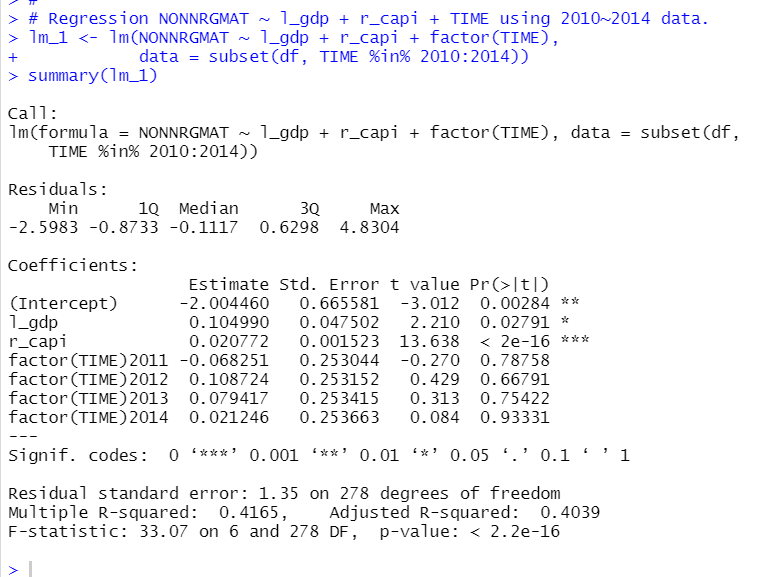p-value for r_capi is almost 0. For TIME variables are not significant. Let's check if there are jointly significant. I use car::linearHypothesis() function.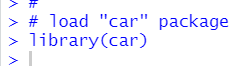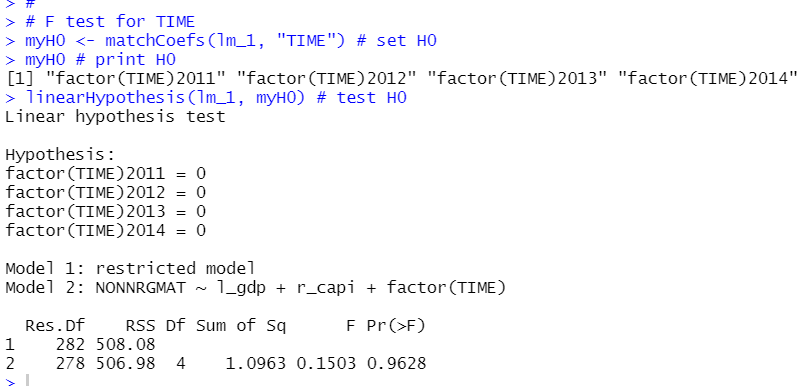I used matchCoef() function to make my Null Hypothesis. Then, I use linearHypothesis() function. The result shows p-value is 0.9628. So, TIME is not jointly significant.

Then, let's check if residuals is Homoskedasticiy or Heteroskedasticiy.
I use bptest() finction in lmtest package.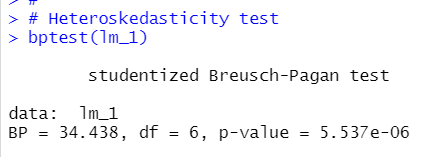p-value is almost 0. So, this regression model has Heteroskedasticiy. Let's confirm lm() function and resid() function.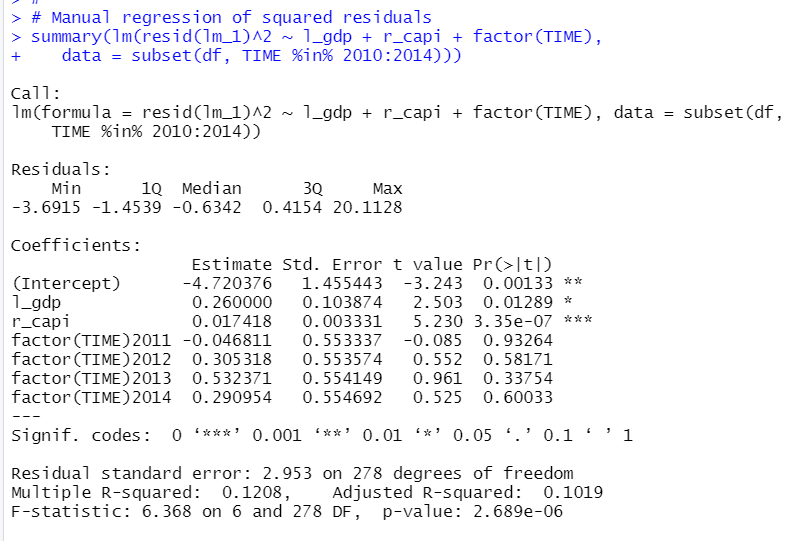p-value is 2.689e-06, it is almost 0. So, we reject Null Hypothesis: residual is Homoskedasticity.

So, I compute Heteroskedasticity robust standard error with lmtest::coeftest( , cvov = hccm) function.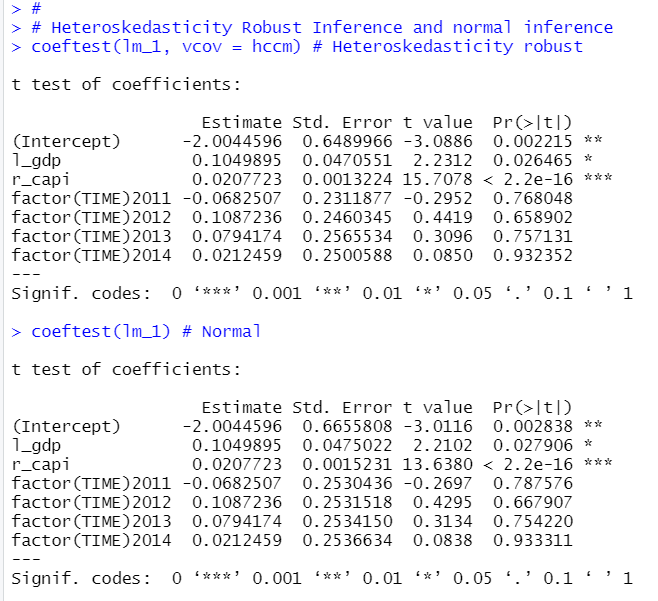The conclusion does not change. l_gdp is significant at 5% level, r_capi is is significant at 0.11% level or below.

Next, let's do Weighted Least Square estimation. I use "weight = 1/r_capi" in lm() function.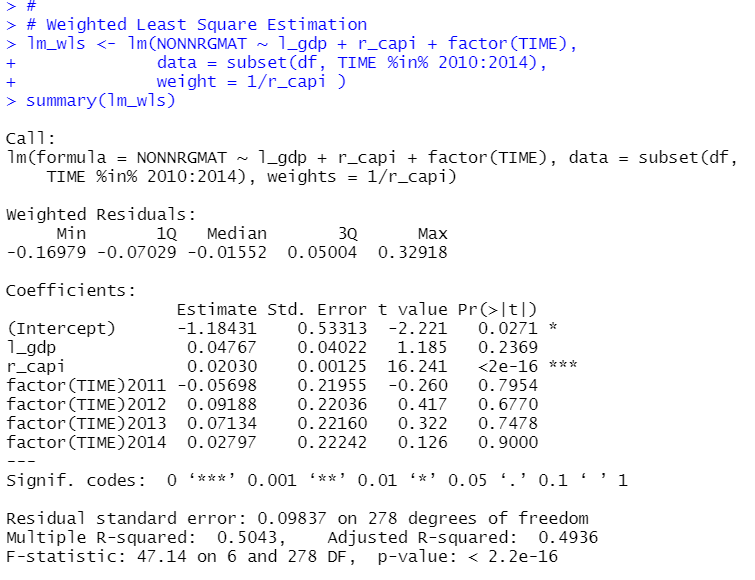Let's compare OLS estimation(lm_1) and WLS estimation(lm_wls) with statgazer package.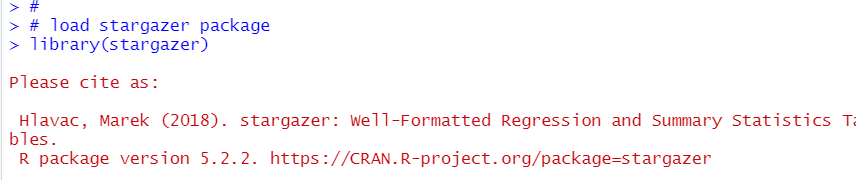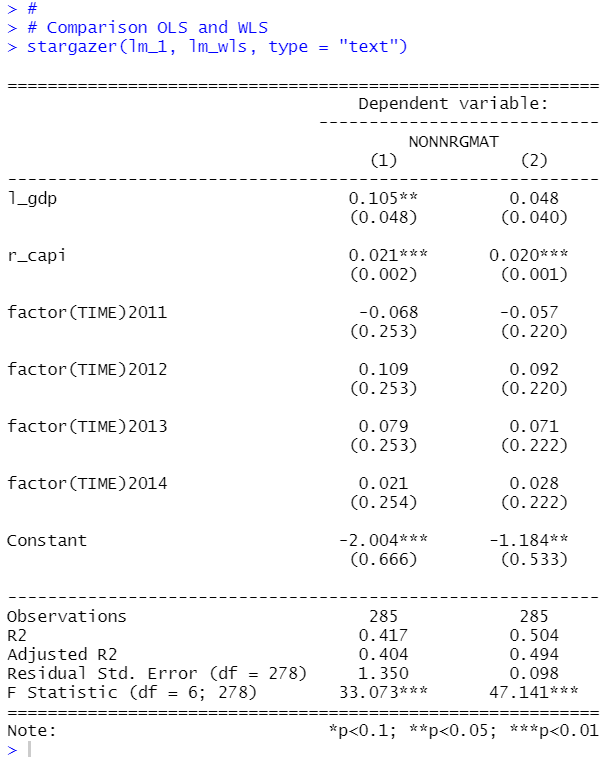(1) is OLS and (2) is WLS. There is not significant change between OLS and WLS for r_capi coefficient.

That's it. Thank you!

Next post it

To read from the 1st post,